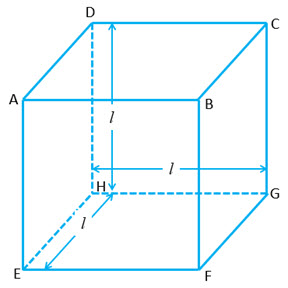Surface Area and Volume of a Cuboid

If the dimensions of the cuboid are: Length$= l$, Breadth$= b$ and Height =$h$. Then:i) Total surface area of the cuboid$= 2(lb + bh + lh)$

ii) Lateral surface are of the cuboid$= 2(l+b)h$

iii) Diagonal of the cuboid$= \sqrt{l^2 + b^2 + h^2}$

iv) Length of all 12 edges of the cuboid$= 4(l + b + h)$

v) Volume of a cuboid$= lbh$

Surface Area and Volume of a CubeIf the length of each of the sides of the cube is$l$. Then

i) Total surface area of the cuboid$= 6l^2$

ii) Lateral surface are of the cuboid$= 4l^2$

iii) Diagonal of the cuboid$= \sqrt{3} l$

iv) Length of all 12 edges of the cuboid$= 12l$

v) Volume of cube$= l^3$